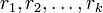# Element structure of alternating groups

This article discusses the element structure of the alternating group$A_n$ of finite degree$n$. Note that for$n = 0,1$, the alternating group coincides with the symmetric group, and for$n = 2$, it is trivial, so the interesting behavior begins from$n = 3$.

The article builds heavily on the element structure of symmetric groups. In particular, we use the fact that cycle type determines conjugacy class in the symmetric group$S_n$.$A_n$ is viewed naturally as the subgroup of$S_n$ comprising even permutations.

## Particular cases$n$ Alternating group Order Element structure page
3 cyclic group:Z3 3 element structure of cyclic group:Z3
4 alternating group:A4 12 element structure of alternating group:A4
5 alternating group:A5 60 element structure of alternating group:A5
6 alternating group:A6 360 element structure of alternating group:A6
7 alternating group:A7 2520 element structure of alternating group:A7
8 alternating group:A8 20160 element structure of alternating group:A8
9 alternating group:A9 181440 element structure of alternating group:A9
10 alternating group:A10 1814400 element structure of alternating group:A10

## Basic facts

### Condition for a permutation to be even

Further information: even permutation

A permutation is an even permutation, i.e., a member of the alternating group, if and only if the number of cycles of even length (which are thus odd cycles) in its cycle decomposition is even. Thus, we can count the number of occurrences of even numbers in the cycle type of a permutation to determine whether the permutation is even. If a permutation is not even, it is odd.

Note that any conjugacy class in$S_n$ is either contained completely in$A_n$ (which means all the permutations are even) or it is contained completely outside$A_n$ (which means all the permutations are odd). This is another way of saying that$A_n$ is a normal subgroup of$S_n$; it is in fact a subgroup of index two and index two implies normal. It is also the kernel of the sign homomorphism that sends even permutations to$+1$ and odd permutations to$-1$.

### Condition for a conjugacy class to split from$S_n$ to$A_n$

Further information: splitting criterion for conjugacy classes in the alternating group

For a conjugacy class of even permutations in$S_n$, the conjugacy class is contained inside$A_n$. There are two possibilities:

1. It remains a single conjugacy class inside$A_n$ (the unsplit case): This happens if it is centralized by some odd permutation. In terms of cycle type, this is equivalent to saying that it either contains a cycle of even length or it contains two cycles of equal odd length.
2. It splits into two conjugacy classes inside$A_n$ (the split case): This happens if its centralizer in$S_n$ is completely contained in$A_n$. In terms of cycle type, this is equivalent to saying that all its cycles have distinct odd length.

Note that when a conjugacy class splits, the two split halves are still automorphic to each other in$A_n$. The conjugation by an odd permutation in$S_n$ restricts to an outer automorphism on the normal subgroup$A_n$.

### Condition for a split conjugacy class in$A_n$ to be real

Further information: criterion for element of alternating group to be real

Any element whose conjugacy class is unsplit from$S_n$, is a real element, and in fact a rational element of$A_n$.

For an element whose conjugacy class is split from$S_n$, the criterion to determine whether it is real or not is as follows: if$r_1, r_2, \dots, r_k$ are the distinct odd cycle lengths of the permutation, it is real if and only if$\sum_{i=1}^k (r_i - 1)/2$ is even. In other words, the number of$r_i$s that are congruent to$3$ modulo$4$ should be even.

## Combinatorics

### Split conjugacy classes

We have canonical bijections:

Conjugacy classes from$S_n$ that split in$A_n$$\leftrightarrow$ Partitions of$n$ into distinct odd parts (via splitting criterion)$\leftrightarrow$ Self-conjugate unordered integer partitions of$n$$\leftrightarrow$ Irreducible representations of$S_n$ that split in$A_n$

For more on these bijections, see set of self-conjugate unordered integer partitions

For this page, we will denote this number by$A$.

### Non-split conjugacy classes

We have equalities:

Number of conjugacy classes of even permutations in$S_n$ that don't split in$S_n$ = Number of conjugacy classes of odd permutations in$S_n$ = Number of (conjugate) pairs of non-self-conjugate partitions of$n$ = Number of pairs (in the sense of restricting to the same thing on$A_n$) of irreducible representations of$S_n$ that don't split in$A_n$.

For this page, we will denote this number by$B$.

### Totals

If$A$ equals the number of conjugacy classes from$S_n$ that split in$A_n$, and$B$ equals the number that don't, then:

• The number of conjugacy classes in$A_n$ is$2A + B$
• The number of conjugacy classes in$S_n$ is$A + 2B$

## Numerical information in particular cases

### Number of equivalence classes of various kinds$n$ Alternating group$A_n$ Order$A=$ number of conjugacy classes from$S_n$ that split in$A_n$$B=$ number of conjugacy classes from$S_n$ that don't split in$A_n$$2A + B =$ number of conjugacy classes in$A_n$$A + 2B =$ number of conjugacy classes in$S_n$$C =$ number of conjugacy classes from$S_n$ which, even after splitting, remain real$2C + B =$ number of conjugacy classes of real elements in$A_n$$A + B + C=$ number of equivalence classes under real conjugacy in$A_n$
3 cyclic group:Z3 3 1 1 3 3 0 1 2
4 alternating group:A4 12 1 2 4 5 0 2 3
5 alternating group:A5 60 1 3 5 7 1 5 5
6 alternating group:A6 360 1 5 7 11 1 7 7
7 alternating group:A7 2520 1 7 9 15 0 7 8
8 alternating group:A8 20160 2 10 14 22 0 10 12
9 alternating group:A9 181440 2 14 18 30 1 16 17
10 alternating group:A10 1814400 2 20 24 42 2 24 24